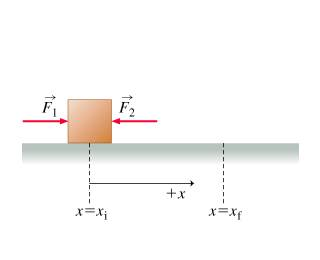Work on Block with an opposing force

Homework Statement

Two forces, of magnitudes F_1 = 75.0 N and F_2 = 20.0 N, act in opposite directions on a block, which sits atop a frictionless surface, as shown in the figure. Initially, the center of the block is at position x_i = -1.00 cm. At some later time, the block has moved to the right, and its center is at a new position, x_f = 2.00 cm.

Find the work W_1 done on the block by the force of magnitude F_1 = 75.0 N as the block moves from x_i = -1.00 cm to x_f = 2.00

Do the same for W_1 by force of magnitude F_2 = 20

Homework Equations

W= F d

Since it's already parallel no need for cos

The Attempt at a Solution

Well, apparently I found out that F * d does not give the correct answer. I also tried subtracting one force or one resultant work from the other but it still isn't correct. The hint that is given to us has something to do with vectors and angles but I don't see how that will apply since the force is already parallel to the surface distance. Is there something hidden that I'm missing? Any thoughts?

Thanks

Hootenanny
Staff Emeritus
Gold Member

Homework Statement

Two forces, of magnitudes F_1 = 75.0 N and F_2 = 20.0 N, act in opposite directions on a block, which sits atop a frictionless surface, as shown in the figure. Initially, the center of the block is at position x_i = -1.00 cm. At some later time, the block has moved to the right, and its center is at a new position, x_f = 2.00 cm.

Find the work W_1 done on the block by the force of magnitude F_1 = 75.0 N as the block moves from x_i = -1.00 cm to x_f = 2.00

Do the same for W_1 by force of magnitude F_2 = 20

Homework Equations

W= F d

Since it's already parallel no need for cos

The Attempt at a Solution

Well, apparently I found out that F * d does not give the correct answer. I also tried subtracting one force or one resultant work from the other but it still isn't correct. The hint that is given to us has something to do with vectors and angles but I don't see how that will apply since the force is already parallel to the surface distance. Is there something hidden that I'm missing? Any thoughts?

Thanks
F.d should work if applied correctly. Perhaps if you showed more detail we could help you out.

Well the only thing I can add on is the diagramOther than that, all that I said is all the information given.

The hint says something like this :

The work W done by a force F_vec in producing a displacement s_vec is given by

W = \vec{F} * \vec{s} = \\vec{F} *\vec{s} *\cos{\phi},
where \vec{F}\ and \\vec{s}\ are the magnitudes of \vec{F} and \vec{s} respectively, and phi is the smaller angle between the two vectors.

The only thing is, I don't see how an angle is applied if the forces are already parallel.
I tried F * d but it says its incorrect.

Hootenanny
Staff Emeritus